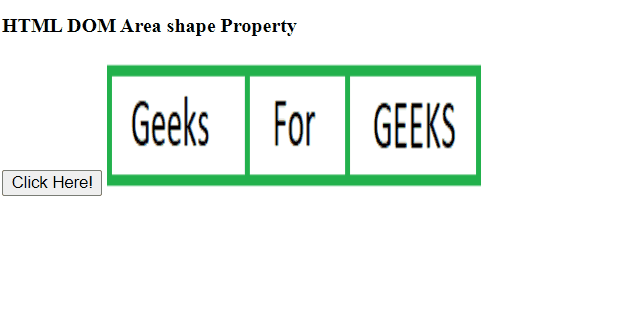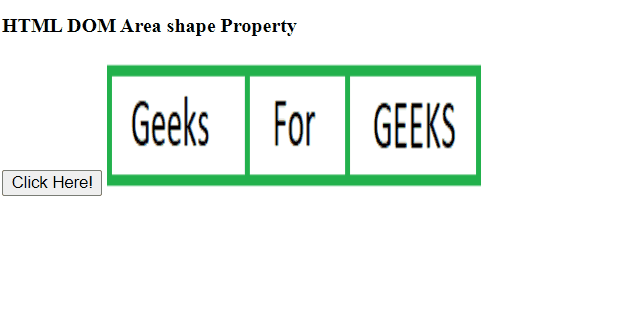# HTML DOM Area shape Property

The Area shape Property in HTML DOM is used to set or return the value of the shape attribute. It is used to define the shape of the area. It is used with coords attribute to define the shape, size, etc.

Syntax:

• It returns the Area shape property.
` areaObject.shape`
• It is used to set the Area shape property.
`areaObject.shape = default|rect|circle|poly `

Property Values: It contains a single value shape which specifies the type of shape.

• default: It is used to specify the entire region.
• rect: It is used to specify the rectangular region.
• circle: It is used to specify a circular region.
• poly: It is used to specify the polygonal region.

Return Value: It returns a string value that represents the shape of the area.

Example 1: This example returns the Area shape Property.

## HTML

 `` `<``html``>`   `<``head``>` `    ``<``title``>` `        ``HTML DOM Area shape Property` `    ``` ``   `<``body``>` `    ``<``h4``> HTML DOM Area shape Property ` `    ``<``button` `onclick``=``"GFG()"``>` `          ``Click Here!` `    ``` `    ``<``map` `name``=``"Geeks1"``>` `        ``<``area` `id``=``"Geeks"` `shape``=``"rect"` `              ``coords``=``"0, 0, 110, 100"` `              ``alt``=``"Geeks"` `              ``href``=` `"https://manaschhabra:manaschhabra499@www.geeksforgeeks.org/"``>` `    ``` `    ``<``img` `src``=` `"https://media.geeksforgeeks.org/wp-content/uploads/a1-25.png"` `         ``width``=``"300"` `height``=``"100"` `         ``alt``=``"Geeksforgeeks"` `         ``usemap``=``"#Geeks1"``>` `    ``<``br``>` `    ``<``p` `id``=``"GEEK!"``>`   `    ``<``script``>` `        ``function GFG() {` `            ``// Return shape property.` `          ``let x = ` `            ``document.getElementById("Geeks").shape;` `            ``document.getElementById("GEEK!").innerHTML = x;` `        ``}` `    ``` ``   ``

Output:Example 2: This example sets the Area shape property.

## HTML

 `` `<``html``>`   `<``head``>` `    ``<``title``>` `        ``HTML DOM Area shape Property` `    ``` ``   `<``body``>` `    ``<``h4``> HTML DOM Area shape Property ` `    ``<``button` `onclick``=``"GFG()"``>Click Here!` `    ``` `    ``<``map` `name``=``"Geeks1"``>` `        ``<``area` `id``=``"Geeks"` `shape``=``"rect"` `              ``coords``=``"0, 0, 110, 100"` `              ``alt``=``"Geeks"` `              ``href``=` `"https://manaschhabra:manaschhabra499@www.geeksforgeeks.org/"``>` `    ``` `    ``<``img` `src``=` `"https://media.geeksforgeeks.org/wp-content/uploads/a1-25.png"` `         ``width``=``"300"` `height``=``"100"` `         ``alt``=``"Geeksforgeeks"` `         ``usemap``=``"#Geeks1"``>` `    ``<``br``>` `    ``<``p` `id``=``"GEEK!"``>`   `    ``<``script``>` `        ``function GFG() {` `            ``// Set shape property.` `           ``let x = ` `            ``document.getElementById("Geeks").shape = "circle";` `            ``document.getElementById("GEEK!").innerHTML =` `                ``"The value of shape attribute has been changed to " + x;` `        ``}` `    ``` ``   ``

Output:Supported Browsers: